## CHAPTER XIIThe Star Group

THIS group comprises those elements known as the inert gases. Their characteristic valence is 0. In the Pendulum diagram they appear on the middle line, alternately with the Bars Group.

Each inert gas has the appearance of a flat six-armed star. All the six arms within one element are the same. Fig. 147.

The arms radiate from a central sphere made of five intersecting tetrahedrons. This sphere first occurs in Neon and is the group Ne120 with which we are familiar. Helium, which is classed by chemists with the inert gases, has a different configuration and has been considered in the Hydrogen Group.

Each member of the Star Group has its meta variety or isotope. On examination of the diagrams it will be seen that in each meta variety each of the six arms has seven more Anu. Therefore the difference between Neon and Meta-Neon is exactly forty-two Anu; and so with all the other elements and their isotopes in the group.

One gas was discovered in the clairvoyant investigations of 1907, for which there is no place in the list of atomic numbers. Its rarity was then described by saying that there might be one in the atmosphere of an ordinary-sized room. It was named by us "Kalon," the "beautiful." and its diagram was published, with that of its meta variety.

 ATOMIC No. ANU ELEMENT CENTRE 6 ARMS 10 360 Neon Ne120 6 [Ne22 + (3Li4) + (2H3)] 10 402 meta-Neon Ne120 6 [Ne22 + mNe15 + I.7 + H3] 18 672 proto-Argon Ne120 6 [N63 + Ne22 + I.7] 18 714 Argon Ne120 6 [N63 + Ne22 + Ar14] 18 756 meta-Argon Ne120 6 [N63 + Ne22 + mNe15 + mAr6] 36 1464 Krypton Ne120 6 [N63 + N110 + Ne22 + mNe15 + Ar14] 36 1506 meta-Krypton Ne120 6 [N63 + N110 + Ne22 + Ne22 + Ar14] 54 2298 Xenon Ne120 6 [Xe15 + Xe14 + N63 + 2N110 + Ne22 + mNe15 + Ar14] 54 2340 meta-Xenon Ne120 6 [2mXe18 + N63 + 2N110 + Ne22 + mNe15 + Ar14] na 3054 Kalon Ne120 6 [Xe15 + Xe14 + 2N63 + 2N110 + 2Ne22 + 2mNe15 + 2Ar14 + Ka12] na 3096 meta-Kalon Ne120 6 [2mXe18 + 2N63 + 2N110 + 2Ne22 + 2mNe15 + 2Ar14 + Ka12] 86 3990 Radon Ne120 6 [Xe15 + Xe14 + 2N63 + 3N110 + 3mNe22 + 3mNe15 + 3Ar14 + I.7] 86 4032 meta-Radon Ne120 6 [Xe15 + Xe14 + 2N63 + 3N110 + 3mNe22 + 3mNe15 + 3Ar14 + I.7 + mRd7]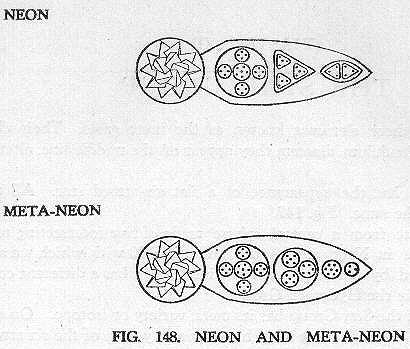FIG. 148. Neon and Meta-Neon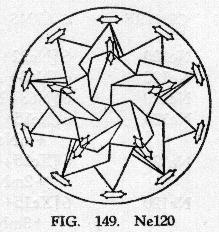FIG. 149. Ne120

• ATOMIC No. 10
• NEON
• As already stated. Neon is in the form of a flat star, with a central globe and six radiating arms. Fig. 148.
• The central globe consists of five interpenetrating tetrahedrons, each tetrahedron being similar to that in Adyarium, Ad24. These five tetrahedrons compose a form which generates the dodecahedron and icosahedron. The group occurs often as the central globe of elements and is distinguished as Ne120. Fig. 149.
• Star. Each arm of the star is composed of three bodies, including one of five spheres, Ne22 which occurs in all the members of this group. Then come three Li4, and finally a group containing two triplets. 2H3.
• Neon = Ne120 + 6(Ne22 + 3Li4 + 2H3)
• Central globe = 120 Anu
• Six arms of 40 Anu = 240
• Total = 360 Anu
• Number weight 360 / 18 = 20.00tk
• Isotope of Neon.
• Meta-Neon differs from Neon by the insertion of an additional Anu in each of the groups included in the second body within its arm, and substituting a group of seven Anu for one of the triplets in the final body. Fig. 148.
• Meta-Neon = Ne120 + 6 [Ne22 + 6mNe15 + I.7 + H3]
• Central globe = 120 Anu
• Six arms of 47 Anu = 282
• Total = 402 Anu
• Number weight 402 / 18 = 22.33tk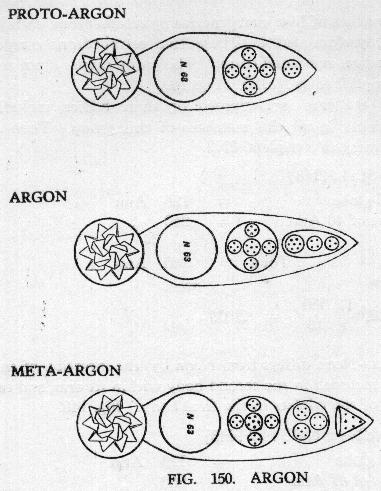FIG. 150. Argon

• ATOMIC No. 18
• ARGON
• The central globe is formed of Ne120.
• Star. Each arm of the star contains the N63 group, then Ne22 and a new group of fourteen Anu, Ar14.
• Argon = Ne120 + 6(N63 + Ne22 + Ar14)
• Central globe = 120 Anu
• Six arms of 99 Anu = 594
• Total = 714 Anu
• Number weight 714 / 18 = 39.666tk
• Meta-Argon.
• This isotope of Argon contains seven more Anu in each arm, the Ar14 being replaced by mNe15 and a cone of six Anu.
• Meta-Argon = Ne120 + 6(N63 + Ne22 + mNe15 + mAr6)
• Central globe = 120 Anu
• Six arms of 106 Anu = 636
• Total = 756 Anu
• Number weight 756 / 18 = 42.00tk
• A curious irregularity appears in Argon. When its weight was determined it was found to be heavier than Potassium instead of being lighter. Argon comes, therefore, out of its proper place in the Periodic Table. But clairvoyant research shows that it does not in reality do so, the true Argon does come in its right place, and its number weight is 37.33. We have called the lighter variety proto-Argon. It is extremely rare in the atmosphere, and the ordinarily known Argon is the commoner variety.
• Proto-Argon Ne120 + 6(N63 + Ne22 + I.7)
• Central globe = 120 Anu
• Six arms of 92 Anu = 552
• Total = 672 Anu
• Number weight 672 / 18 = 37.33tk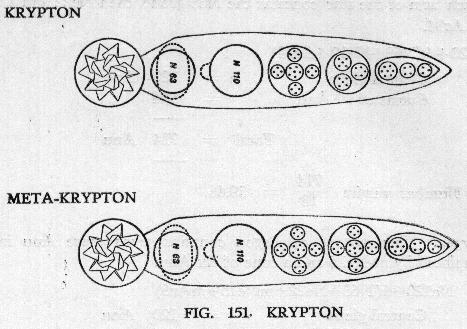FIG. 151. Krypton

• ATOMIC No. 36
• KRYPTON
• Central globe. As in all the Star Group elements the central globe is Ne120.
• Star. Each arm of the star contains constituents from Argon and Meta-Argon, with the addition of an N110. The groups N110 and N63 appear constantly in the building up of these elements. When these two bodies appear one above the other there is a strong attraction between them; the sphere-wall of N110 is pulled towards N63, while the sphere wall of the latter undergoes a flattening compression.
• Krypton = Ne120 + 6 (N63 + N110 + Ne22 + mNe15 + Ar14)
• Central globe = 120 Anu
• Six arms of 224 Anu = 1344
• Total = 1464 Anu
• Number weight 1464 / 18 = 81.33tk
• Meta-Krypton.
• This isotope is slightly higher in atomic weight, and the two together should make up about the atomic weight given by science.
• It differs from Krypton only in the substitution of Ne22 for mNe15 in each arm of the star.
• Meta-Krypton = Ne120 + 6 (N63 + N110 + 2Ne22 + Ar14)
• Central globe = 120 Anu
• Six arms of 231 Anu = 1386
• Total = 1506 Anu
• Number weight 1506 / 18 = 83.666tk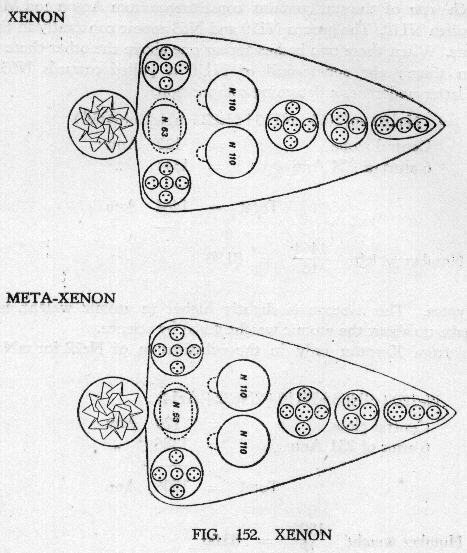FIG. 152. Xenon

• ATOMIC No. 54
• XENON
• The central globe is Ne120.
• Star. Each arm of the star contains the constituents of Krypton, with the addition of another N110 globe and two smaller spheres, Xe14 and Xe15. These are arranged symmetrically as shown in Fig. 152.
• Xenon Ne120 + 6[Xe15 + Xe14 + N63 + 2N110 + Ne22 + mNe15 + Ar14]
• Central globe = 120 Anu
• Six arms of 363 Anu = 2178
• Total = 2298 Anu
• Number weight 2298 / 18 = 127.666tk
• Meta-Xenon.
• Again the isotope, being of higher atomic weight, would make the mean value for the element approach that of science.
• It differs from Xenon in the substitution of the two bodies. 2Xe18 for Xe15 and Xe14, thus making up the difference of seven Anu.
• Meta-Xenon Ne120 + 6(2mXe18 + N63 + 2N110 + Ne22 + mNe15 + Ar14)
• Central globe = 120 Anu
• Six arms of 370 Anu = 2220
• Total = 2340 Anu
• Number weight 2340 / 18 = 130.00tk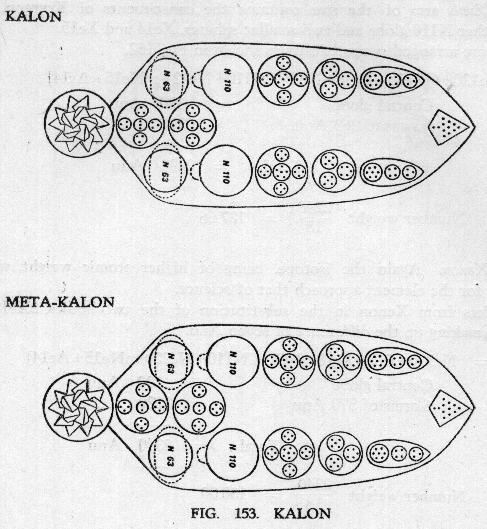FIG. 153. KALON

• ATOMIC No. na
• KALON
• The central globe is, as usual, Ne120.
• Star. The arms are now much more complex. Kalon contains twice the constituents of Krypton, with the addition of Xe14 and Xe15 from Xenon and a curious cone. Ka12, possessing a kind of tail. Fig. 153.
• Only a few atoms of Kalon and Meta-Kalon have been found in the air of a fairsized room. This probably accounts for the fact that they have not yet been isolated by science.
• Kalon = Ne120 + 6(Xe15 + Xe14 + 2N63 + 2N110 + 2Ne22 + 2mNe15 + 2Ar14 + Ka12)
• Central globe = 120 Anu
• Six arms of 489 Anu = 2934
• Total = 3054 Anu
• Number weight 3054 / 18 = 169.666tk
• Meta-Kalon.
• The isotope contains seven extra Anu, made up, as in the case of Xenon, by the substitution of two mXe18 for Xe15 and Xe14.
• Meta-Kalon = Ne120 + 6 [2mXe18 + 2N63 + 2N110 + 2Ne22 + 2mNe15 + 2Ar14 + Ka12]
• Central globe = 120 Anu
• Six arms of 496 Anu = 2976
• Total = 3096 Anu
• Number weight 3096 / 18 = 172.00tk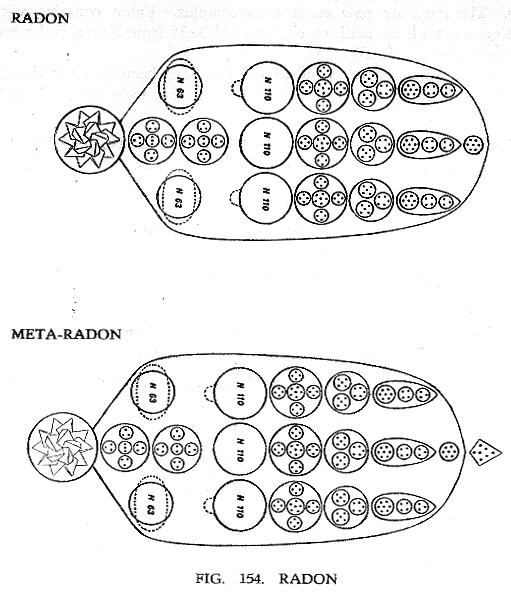• ATOMIC No. 86
• Scientists place Radon in this group of the inert gases. It was first known as Radium Emanation and is formed by the action of the powerful vortex of Radium. The central globe is, as usual, Ne120.
• Star. The six arms each contain three columns.
• Radon = Ne120 + 6[Xe15 + Xe14 + 2N63 + 3N110 + 3mNe22 + 3mNe15 + 3Ar14 + I.7]
• Central globe = 120 Anu
• Six arms of 645 Anu = 3870
• Total = 3990 Anu
• Number weight 3990 / 18 = 221.666tk
• The metes variety of Radon is extremely rare. It is also noteworthy for the irregularity that in its metes-variety each arm has the extra seven Anu outside the arm, and not within it.
• Metes-Radon = Ne120 + 6(Xe15 + Xe14 + 2N63 + 3N110 + 3mNe22 + 3mNe15 + 3Ar14 + I.7 + mRn.7)
• Central globe = 120 Anu
• Six arms of 652 Anu = 3912
• Total = 4032 Anu
• Number weight 4032 / 18 = 224.00tk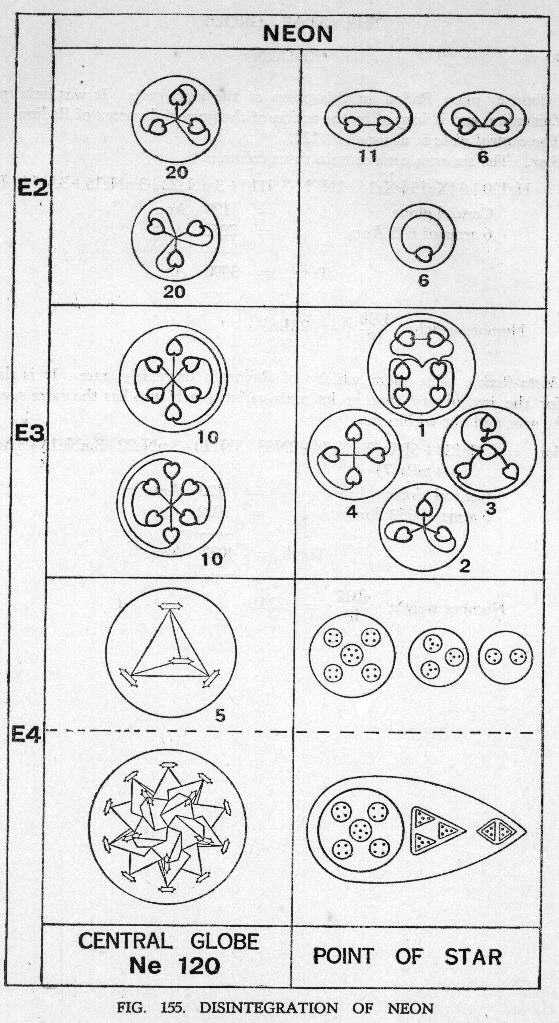FIG. 155. DISINTEGRATION OF NEON

### DISINTEGRATION OF THE STAR GROUP

• DISINTEGRATION OF NEON
• In the first stage of the disintegration of Neon on the E4 level the star gives its central globe and six ovoids from the six points of the star. The globe, Ne120, then breaks up further, giving its five tetrahedrons Ad24. The ovoids each liberate three spheres containing 22, 12 and 6 Anu.
• On the E3 level the Ad24 each give four Ad6, and the spheres a sestet, four quartets of a cross type, three quartets of the pyramid type and two triads.
• On the E2 level the Ad6 each give two triplets and the other groups break up into duads and units.
• Many of the component parts of the elements in this group are familiar and their disintegration may be followed under other elements.

Fig. 156 shows the elements of the Star Group in a condensed form, from which their relationships can be studied.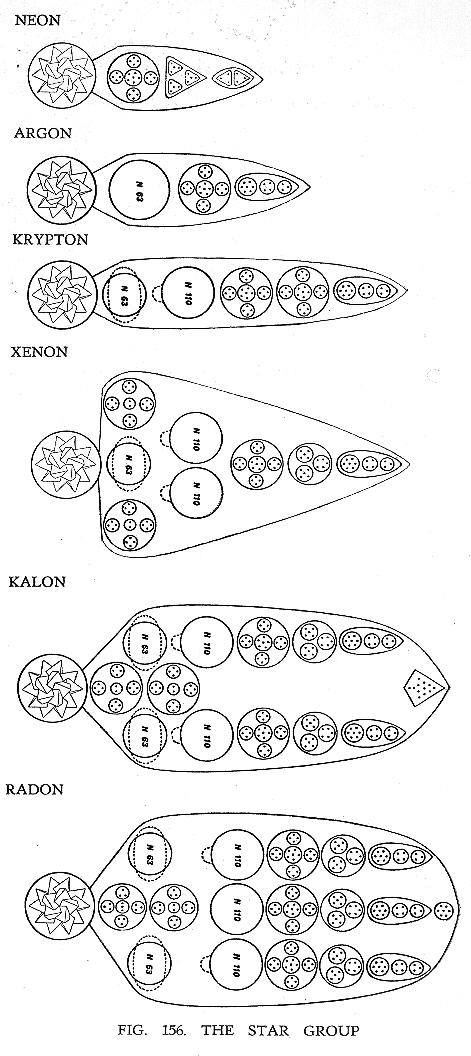FIG. 156. THE STAR GROUP

FIG. 157. THE MOLECULE OF WATER, H2O.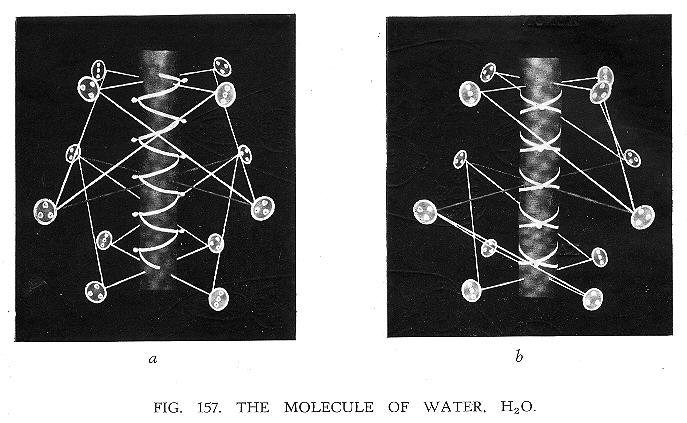Next: Chapter XIII   Compounds

## Acknowledgements

 TPH Twilight Archive

HTML validation by:

 W3C online validation service for HTML 4.0

spell-checked, files merged, some errors corrected,
reset in HTML 4.0 in March, April, May 2000
last revision November 2003